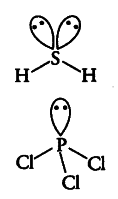# Explain the non-linear shape of \$H _{ 2 }S\$ and non-planar shape

Explain the non-linear shape of \$H { 2 }S\$ and non-planar shape of
\${ PCl }
{ 3 }\$ using valence shell electron pair repulsion theory.

\$H _{ 2 }S\$—In it the central atom is sulphur. There are 6 electrons in its valence shell (16S= 2,8,6). Two
electrons are shared with two H-atoms and the remaining four electrons are present as two one pair. Hence, total pairs of electrons are four (2 bond pairs and 2 lone pairs). Due to the presence of 2 lone pairs the shape becomes distorted tetrahedral or angular or bent (non-linear).{ PCl }_{ 3 } — In it the central atom is phosphorus. There are 5 electrons in its valence shell (15 P = 2, 8, 5). Three electrons are shared with three Cl-atoms and the remaining two electrons are present as one lone pair. Hence, total pairs of electrons are four (1 lone pair and 3 bond pairs). Due to the presence of one lone pair, the shape becomes pyramidal (non-planar).